# SQL ALTER TABLE

## ALTER TABLE

The SQL ALTER TABLE command is used to change the structure of an existing table. It helps to add or delete columns, create or destroy indexes, change the type of existing columns, or rename columns or the table itself.

It can also be used to change the comment for the table and type of the table.

Syntax:

```ALTER TABLE <table_name>(
...);
```

Parameters:

Name Description
table_name Name of the table where data is stored.
column1,column2 Name of the columns of a table.
data_type Char, varchar, integer, decimal, date and more.
size Maximum length of the column of a table.

## SQL ALTER TABLE statement to add a column to a table

In the following topic, we are discussing the SQL ALTER TABLE statement, which adds a column to a table. If not specified otherwise, the column will be added at the end of the table.

Sample table: agent1

To add a new column 'email' at the end of the table 'agent1' with field name and data type

Field Name Data Type Size Decimal Places NULL Constraint
e-mail char 25   No

the following SQL statement can be used :

SQL Code:

``````ALTER TABLE agent1 ADD email char(25);
``````

Output: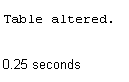To see the modified structure of the said table:

SQL Code:

``````DESCRIBE agent1;
``````

## Structure## SQL ALTER TABLE statement to drop a column

In the following example, we are discussing, how a column can be dropped from a table if it exists in the table, using the SQL ALTER TABLE statement.

Sample table: agent1

To drop the existing column 'country' from the table 'agent1', the following SQL statement can be used:

SQL Code:

``ALTER TABLE agent1 DROP(country); ``

Output: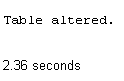To see the modified structure of the said table:

SQL Code:

``````DESCRIBE agent1;
``````

## Structure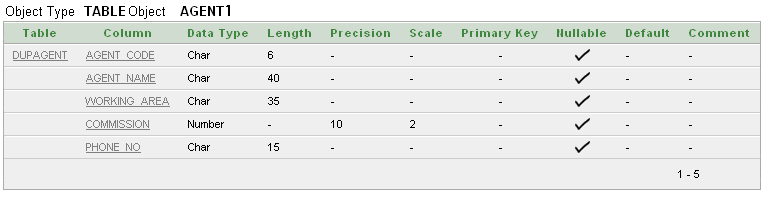## SQL ALTER TABLE statement to change or drop default value of a column

In the following example, we are going to discuss how to drop the default value of a column from a table if the column exists in the table using SQL ALTER TABLE statement.

Example:

Sample table: agent1

To modify the existing column 'commission' of 'agent1' table with a default value .05,

the following SQL statement can be used:

SQL Code:

``````ALTER TABLE agent1
MODIFY commission DEFAULT .05;
``````

Output:To see the modified structure of the said table :

SQL Code:

``````DESCRIBE agent1;
``````

## Structure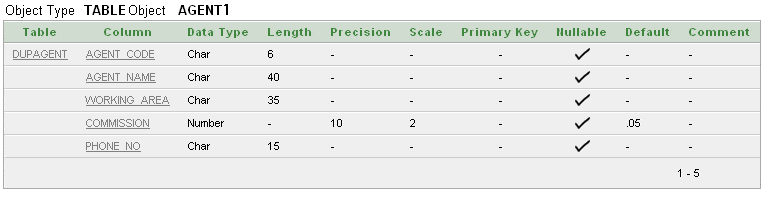## SQL ALTER TABLE statement to drop default value of a column

To drop the existing default value of column 'commission' of 'agent1' table, the following SQL statement can be used:

SQL Code:

``````ALTER TABLE agent1
MODIFY commission NUMBER;
``````

Output: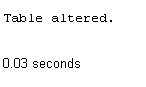## SQL ALTER TABLE statement to add individual column constraint

In the following example, we are going to discuss about the usage of SQL ALTER TABLE statement to add a constraint for a column and also drop an existing constraint of a column from a table if the column exists in the table.

Sample table: agent1

To add a UNIQUE CONSTRAINT named 'dup_che_con' for the the existing column 'agent_code' of 'agent1' table,

the following SQL statement can be used:

SQL Code:

``````ALTER TABLE agent1 ADD CONSTRAINT
dup_che_con UNIQUE(agent_code);
``````

Output:## SQL ALTER TABLE statement to drop individual column constraint

To drop the existing UNIQUE CONSTRAINT 'dup_che_con' from the table 'agent1',

the following SQL statement can be used:

SQL Code:

``````ALTER TABLE agent1
DROP CONSTRAINT dup_che_con;
``````

Output:## SQL ALTER TABLE statement to change size and data type of a column

In the following example, we are going to discuss about the usage of SQL ALTER TABLE statement to change the size and data type of a column in an existing table, if the column exists in the table.

Example:

Sample table: agent1

To modify the datatype and size of the column 'country' of 'agent1' table, the following SQL statement can be used:

SQL Code:

``````ALTER TABLE  agent1
MODIFY (country VARCHAR2(35));
``````

Output: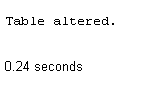## SQL ALTER TABLE statement to add or drop PRIMARY KEY of a table

In the following example, we are going to discuss about the usage of SQL ALTER TABLE statement to add and drop primary key of a table.

Sample table: agent1

To add a PRIMARY KEY CONSTRAINT named 'pk_ag_code' for the column 'agent_code' of the 'agent1' table, the following SQL statement can be used:

SQL Code:

``````ALTER TABLE  agent1
PRIMARY KEY(agent_code);
``````

Output:## SQL ALTER TABLE statement to drop existing PRIMARY KEY of a table

To drop the existing PRIMARY KEY CONSTRAINT named 'pk_ag_code' form the 'agent1' table, the following SQL statement can be used:

SQL Code:

``````ALTER TABLE  agent1
DROP CONSTRAINT pk_ag_code;
``````

Output:## SQL ALTER TABLE statement to add or drop FOREIGN KEY of a table

In the following example, we are going to discuss about the usage of SQL ALTER TABLE statement to add and drop foreign key of a table.

Sample table: customer1

To add a FOREIGN KEY CONSTRAINT named 'fk_ag_code' for the column 'agent_code' of the 'customer1' table, the following SQL statement can be used:

SQL Code:

``````ALTER TABLE customer1
fk_ag_code
FOREIGN KEY (agent_code)
REFERENCES agents(agent_code);
``````

Output:## SQL ALTER TABLE statement to drop existing FOREIGN KEY of a table

To drop the existing FOREIGN KEY CONSTRAINT named 'fk_ag_code' form the 'customer1' table, the following SQL statement can be used :

SQL Code:

``````ALTER TABLE  customer1
DROP CONSTRAINT fk_ag_code;
``````

Output:## SQL ALTER TABLE statement to add CHECK CONSTRAINT

In the following example, we are going to discuss about the usage of SQL ALTER TABLE statement to add and drop CHECK CONSTRAINT of column(s) of a table.

## Sample table : customer1

To add a CHECK CONSTRAINT named 'du_che_con' for the column 'grade' of the 'customer1' table, which checks whether the values of 'grade' are within the range 1 to 3 at the time of inserting rows into the table, the following SQL statement can be used :

SQL Code:

``````ALTER TABLE customer1
du_che_con
``````

Output: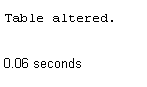## SQL ALTER TABLE statement to drop CHECK CONSTRAINT

To drop the existing CHECK CONSTRAINT named 'du_che_con' form the 'customer1' table, the following SQL statement can be used :

SQL Code:

``````ALTER TABLE customer1
DROP CONSTRAINT du_che_con;
``````

Output: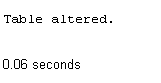## SQL ALTER TABLE statement to change PRIMARY KEY CONSTRAINT

In the following example, we are going to discuss about the usage of SQL ALTER TABLE statement to modify the PRIMARY KEY and FOREIGN KEY constraint.

To modify a PRIMARY KEY and FOREIGN KEY constraint, firstly it is needed to remove the existing PRIMARY KEY and FOREIGN KEY constraint and then re-create it.

Sample table : agent1

Suppose there is a PRIMARY KEY CONSTRAINT named 'pk_ag_code' for the column 'agent_code' of the 'agent1' table.

To modify the PRIMARY KEY CONSTRAINT named 'pk_ag_code, the following SQL statements can be used :

SQL Code:

``````ALTER TABLE  agent1
DROP CONSTRAINT pk_ag_code;
``````

SQL Code:

``````ALTER TABLE  agent1
PRIMARY KEY(agent_code);
``````

## SQL ALTER TABLE statement to change FOREIGN KEY CONSTRAINT

Sample table: customer1

Suppose, there is a FOREIGN KEY CONSTRAINT named 'fk_ag_code' for the column 'agent_code' of the 'customer1' table

To modify the FOREIGN KEY CONSTRAINT named 'fk_ag_code', the following SQL statements can be used :

SQL Code:

``````ALTER TABLE customer1
DROP CONSTRAINT
fk_ag_code;
``````

SQL Code:

``````ALTER TABLE customer1
FOREIGN KEY (agent_code)
REFERENCES agents(agent_code);
``````

Outputs of the said SQL statement shown here is taken by using Oracle Database 10g Express Edition.

Practice SQL Exercises

Previous: Constraints
Next: SELECT Statement

﻿

## SQL: Tips of the Day

What is the simplest SQL Query to find the second largest value?

```SELECT MAX(col)
FROM table
WHERE col NOT IN ( SELECT MAX(col)
FROM table
);
```

Ref: https://bit.ly/3jPa662